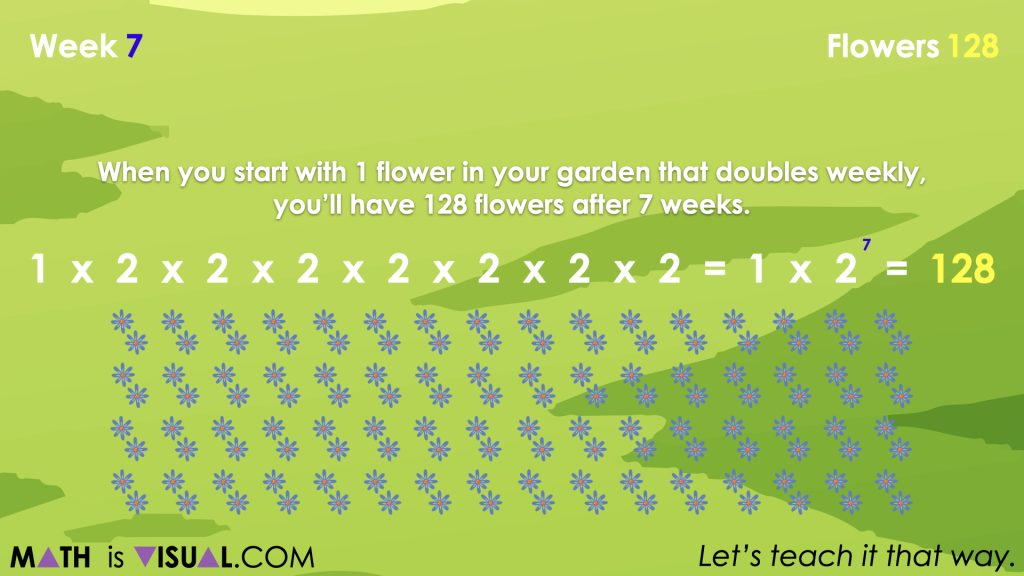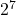# Visualizing Powers Through Exponential Growth Patterns

Investigate subtracting negative numbers by finding the difference in temperature using the thermometer as a linear model number line.

## In This Set of Visual Number Talk Prompts…

Students will extend patterns with exponential growth, make near and far predictions about the pattern, describe the pattern in words, and determine the general term.

## String of Related Problems

The following visual number talk is a set of visual patterns that you will share with students one at a time. In order to build on the context from day 1, we will be using the context of weeds (or flowers) growing in a garden. Ask students to describe the change. Collaboratively make a generalization about the relationship between the independent variable, x, and the dependent variable, y, in order to create an exponential general term, written as an expression or as an equation, depending on which you prefer to emerge. Use the exponential expression or equation to determine the value of the 7th term for each exponential relationship.

## Visual Math Talk Prompt #1

Students will be prompted with:

How many flowers will there be in the garden by week 7?Students will likely recognize that beginning in week 0, the garden began with 1 flower, then doubled each week afterwards.

By continuing this pattern, students will continue doubling as each week passes until reaching 128 flowers in week 7.Be sure to highlight that since we are doubling each week, we can simply take our original number of flowers (1) and multiply by 2 a total of 7 times – once for each of the 7 passing weeks.

We can write this as:

1 x 2 x 2 x 2 x 2 x 2 x 2 x 2

= 1 x= 128

Therefore, you should expect 128 flowers by week 7.

## Want to Explore These Concepts & Skills Further?

Two (2) additional number talk prompts are available in Day 2 of the Invasive Species problem based math unit that you can dive into now.

Why not start from the beginning of this contextual 5-day unit of real world lessons from the Make Math Moments Problem Based Units page.

Did you use this in your classroom or at home? How’d it go? Post in the comments!

Did you use this in your classroom or at home? How’d it go? Post in the comments!

Math IS Visual. Let’s teach it that way.